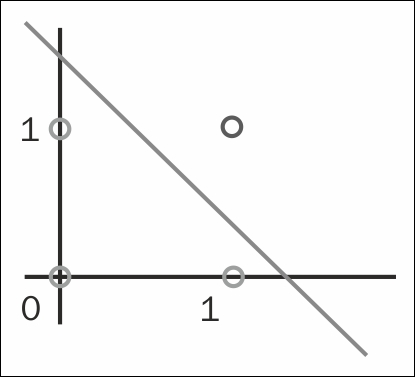## Creating a neural network perceptron

A perceptron is a linear classifier that uses labelled data to converge to its answer. Given a set of inputs and their corresponding expected output, a perceptron tries to linearly separate the input values. If the input is not linearly separable, then the algorithm may not converge.

In this recipe, we will deal with the following list of data:

[(0,0), (0,1), (1,0), (1,1)].

Each item is labelled with an expected output as follows:

• `(0,0)` is expected to output a `0`

• `(0,1)` is expected to output a `0`

• `(1,0)` is expected to output a `0`

• `(1,1)` is expected to output a `1`

Graphically, we are trying to find a line that separates these points:1. Import `replicateM`, `randomR`, and `getStdRandom...`